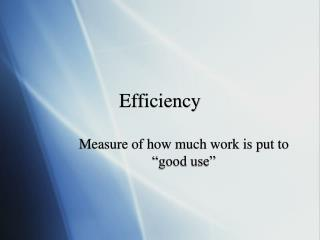DownloadDownload PresentationEfficiency

Efficiency

Télécharger la présentationEfficiency

- - - - - - - - - - - - - - - - - - - - - - - - - - - E N D - - - - - - - - - - - - - - - - - - - - - - - - - - -
Presentation Transcript

1. Efficiency Measure of how much work is put to “good use”

2. Efficiency of Machines • Law of C of E says that energy in must equal energy out • However, often a lot of energy is lost • Heat, friction, sound, etc. • Efficiency equals • (Useful energy out) *100% (Energy in) • Higher the percentage….the more efficient the machine is

3. Efficiency (cont.) • Companies seek to find most efficient machines to manufacture, transport, and develop • Ex. Gas powered cars are not very efficient, about 10 - 25% efficient • Electric (hybrid) cars--much more efficient • Get up to 3 times the mileage of some gas cars • http://auto.howstuffworks.com/hybrid-car4.htm • Government standards

4. 100% Efficient

5. Problem • http://www.bbc.co.uk/schools/gcsebitesize/physics/energy/energyefficiencyrev3.shtml

6. Question 1 • A power plant burns 75kg of coal every second. Each kg of coal contains 27 MJ (27 million joules) of chemical energy. • What is the energy output of the power station every sec? The Solution • = 75 x 27 million J per sec • = 2025 million J per sec • = 2025 million J/s or • (2025 megaWatts)

7. Question 2 • The electrical power output of the power plant is 800MW (800 million watts). But Question 1 stated that the chemical energy output of the station was 2025 MW…..So, What has happened to the rest of the energy? The Answer • Most of the rest of the energy is wasted as heat - up the chimney of the power station, in the cooling towers, and because of friction in the machinery.

8. Question 3 • Calculate the efficiency of the power plant as a percentage. The Solution • Efficiency = useful power output/total power input • = 800,000,000 W/2025,000,000 W • = 0.395 x 100% to create a percentage • = 39.5%

9. Simple Machines • wedge, pulley, lever, ramp, screw, wheel and axle • Multiply force but applying small force over greater distance • Amount of work done is not increased by a machine • By law of conservation of energy it is impossible to multiply energy A screw applies a small force over the long distance across the face of all of its threads at once to accomplish the same work as a large force over a small distance

10. Mechanical Advantage By applying a 10 N force and moving this end 50 cm.. • Usefulness of machines is due to multiplication of force, not of energy • Often limited by how much force we can apply, so we apply a small force over a large distance • One way to measure how useful a simple machine is is by measuring its Ideal Mechanical Advantage • MA = Force output / force input Soo….MA = 10/1 = 10 You were able to apply a 100 N force on this end and move the heavy rock 5 cm

11. Incline Plane • MA is made up by comparing the Parallel force by the force of gravity, on any incline the parallel force will be much less than the weight of the object, this is why it is easier to walk long distance on low incline than a short distance and a steep incline or climbing straight up

12. Schober Brothers embark on a hike in Yosemite Ntnl. Park This is easy!! Idiots! Day 1, short hike…little wet…. feelin good. …..And learn a harsh lesson about Incline Planes…

13. Day 2 Hike -- 2600 ft of vertical climb to top of Yosemite Falls 2600 vertical feet

14. Hike on Day 2-- In pain, not even close to the top

15. Me slumped on a rock about to vomit…. …………………Will cant feel his legs Andy takes picture and laughs Little higher up the mountain….

16. Get to the top ..Exhausted…. Pass out on a rock while squirrel eats our granola

17. The Point…

18. The Point…. (cont.) • The steeper the incline plane… the higher portion of your weight you are going to have to lift with every step. • Low incline, medium incline, direct climb all require the same amount of work because all produce same increase in PE • Difference is in how that work is performed.

19. Pulleys • Like levers, ramps, and screws…. Sacrifices displacement to achieve a greater force • By pulling a greater displacement you have to apply less force • MA is shown by how many ropes are supporting the load in this case there are two • http://en.wikipedia.org/wiki/Pulley

20. Another Pulley • MA = 4 • 4 ropes supporting load • Force applied is 4 times less than 100 N • So rope must be pulled with 25 N of force with a distance 4 times greater than the upward distance the load moves

21. Levers • Pull greater distance on long end but achieve greater force over a small distance on the short end